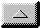Analytical TheoryLinear shallow-water wave theory applied to step topography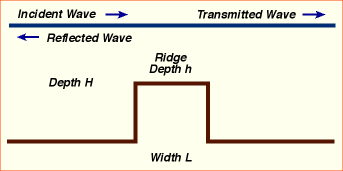A planar monochromatic wave, hI incident on a ridge, produces a transmitted wave, hT and a reflected wave, hRThe dispersion relation is w / k = c where c =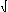(gh) for normal incidence The transmissivity is defined as the ratio of amplitudes T = | AT | / | AI |Effects of Cross-Ridge Phase q = kL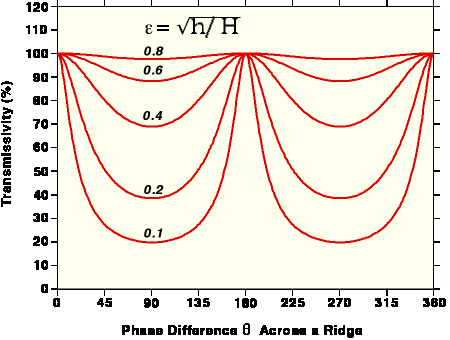Minimum transmission (Tmin) occurs when q = 90°, 270°, 450°,... 100% transmission occurs when the cross-ridge phase shift q = kL is exactly q = 180°, 360°,...Effects of Ridge Depth on TransmissivityThe actual transmissivity is close to Tmin when q < 90° , i.e. for ridges that are narrower than a quarter wavelength as computed from the ridge-top depth hAt higher frequencies w, rapid fluctuations occur between and T=Tmin << 100% as w varies (T~100% when e << 1 is a highly idealized result)Smoothing Smith/Sandwell Topography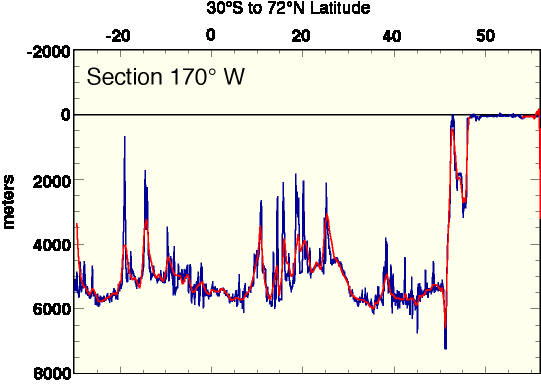•   2-D orthogonal wavelet transform (LA8) was performed on the gridded topography•   The shorter-scale wavelet amplitudes were then set to zero with a tapered cutoff in scale •   Finally, the amplitudes were back-transformed to produce the smoothed topography